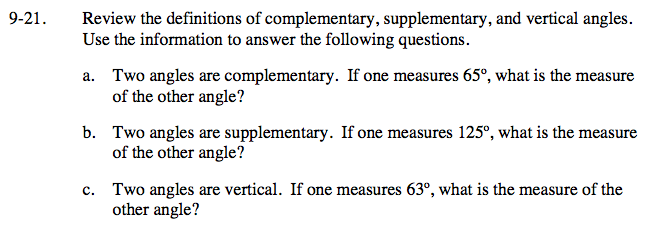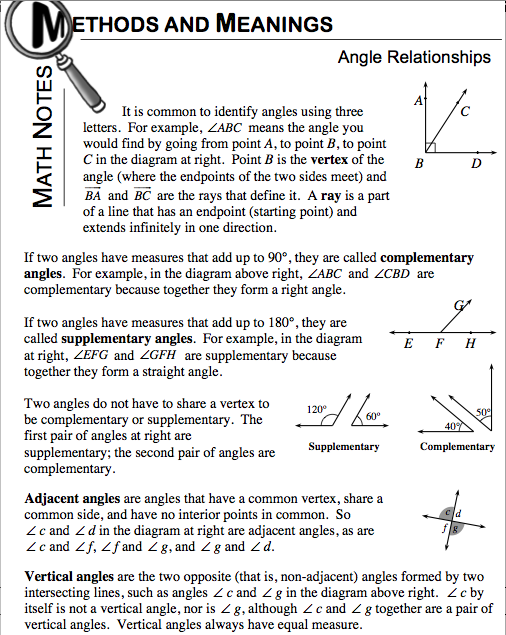Home > ACC7 > Chapter cc29 > Lesson cc29.1.1 > Problem9-21

9-21.
1. Review the definitions of complementary, supplementary, and vertical angles. Use the information to answer the following questions. Homework Help ✎

1. Two angles are complementary. If one measures 65º, what is the measure of the other angle?

2. Two angles are supplementary. If one measures 125º, what is the measure of the other angle?

3. Two angles are vertical. If one measures 63º, what is the measure of the other angle?Refer to the Math Notes Box from Lesson 8.3.2 to help you answer this question.1 2